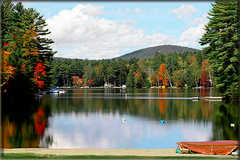Alchemists Looked to Neolithic Number SquaresAlchemists Looked to Neolithic Number Squares. Neolithic and even earlier Mesolithic builders took their inspiration from number squares. They placed their characteristic numbers into foundations. I’ve blogged about Lepenski Vir.Here is an excerpt from one:Lepenski Vir is Proof of the use and understanding of an Ancient Number Square.  A Mesolithic site on the Blue Danube used the codes of the square of three.  Lepenski Vir (Лепенски Вир, Lepen Whirl) is an important Mesolithic archaeological site located in Serbia in central Balkan peninsula The latest radiocarbon and AMS data suggests that the chronology of Lepenski Vir is compressed between 9500/7200-6000 BC.The dimensions of Lepenski Vir prove Mesolithic knowledge of the 3 x 3 number square’s hidden codes.

The entire foundation area  is framed by a roughly rectangular shape frame of 185 x 55 yards. Over 1000’s of years the same dimensions have been used for many construction projects.  Some examples:

Here is some samples of how to find the 555 code: Take two sets of three opposite numbers. Add them together. Then divide them by two for an average: Examples are 492 + 618 = 1110. Divided by two = 555. Another example, 834 + 276 = 1110, Divided by two = 555. The middle 951 is added to itself backwards as 159 + 951 = 1110. Divided by 2 = 555. Ths simple number square is also the most complex. The builders of its ancient foundation in Yugoslavia totally understood this. Now for some mathematical fun:

ALCHEMISTS USED NUMBER SQUARES TO TURN LEAD INTO GOLD

Here’s what the alchemists thought of number squares and of this square in particular : (1) They  unified all the sciences and arts. (2) They were thought to hold  the elusive secret of turning lead into gold.

• Each of the 7 known planets in antiquity was represented by the banner of a number square and a metal. Saturn’s was the featured 3 x 3 and metal, lead . The Sun was the 6 x 6 and gold.
• The Sun, square (gold)  totalled numbers 1 through 36. Add these numbers 1 + 2 + 3 …through  36. They total the Biblical 666. Saturn’s (lead) were 1 – 9. They total 45. This is 621 less than the other total However:
• Let’s add the alchemical fusion of numbers to the square of Saturn. We will consider the numbers three at the time. This will be done vertically and horizontally.

(1) horizontally: 492 + 618 = 1110.    816 + 294 = 1110.  Any number that crosses the cenral three at the time is its own number backwards. (This is so much like the triplet codons of the RNA-DNA code.) Thus, 357 + 753 = 1110, The horizontal total is 3330. Now let’s look at the vertical total:

(2) Vertically: 438 + 672 = 1110. 276 + 834 = 1110,   951 + 159 = 1110. The vertical total is 3330.

(3) Finally, add vertical and horizontal totals together as the alchemists did: 3330 + 3330 = 6660. Zero was a synthetic number in antiquity. It was formed by the combination of any two opposite numbers on the 3 x 3 square. The numbers on the square were the 9 prime numbers.  Ten was a synthetic number combination as 9 + 1; or 3 + 7 etc. Ten was not an entity in and of itself. Thus, by the association with the not so obvious code of the square of three of Saturn; we have just equated it with the  numerical properties of the square of the Sun by common number.  We have just turned the lead of Saturn into the gold of the Sun. Most certainly, this is a wonderful symbolic thought. It is a refinement  of baser qualities (lead) into higher and noble qualities (gold) .Oquaga Lake where I learned about number squares from a spirit.